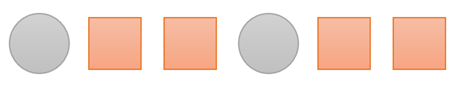Mathematics
Easy

Question

# What is the 20th shape? The rule is “Circle, Square, Square.”## Square    Circle    Rectangle    TriangleHint:

## The correct answer is: Square

### The given pattern in words is Circle, Square, Square.We are asked to find the shape at the 20th place.The rule used to write the pattern is “Circle, Square, Square”A single unit of is repeating. The unit is of Circle, Square, Square. The last shape of the unit is Square. The unit has three shapes.So, all multiples of three will have Square in their position.We have to find the shape at 20th place. It is not a multiple of 3The number closest to 20, which is a multiple of 3 is 18. It is also less than 20. So, at 18th position there will be Square.20 is two places after 18. The shape which will come two places after last Square is again a Square.So, the shape at the 20th place is a Square.

While solving such questions, we should be careful about the order of the shapes.

### Related Questions to study#### With Turito Foundation.#### Get an Expert Advice From Turito.